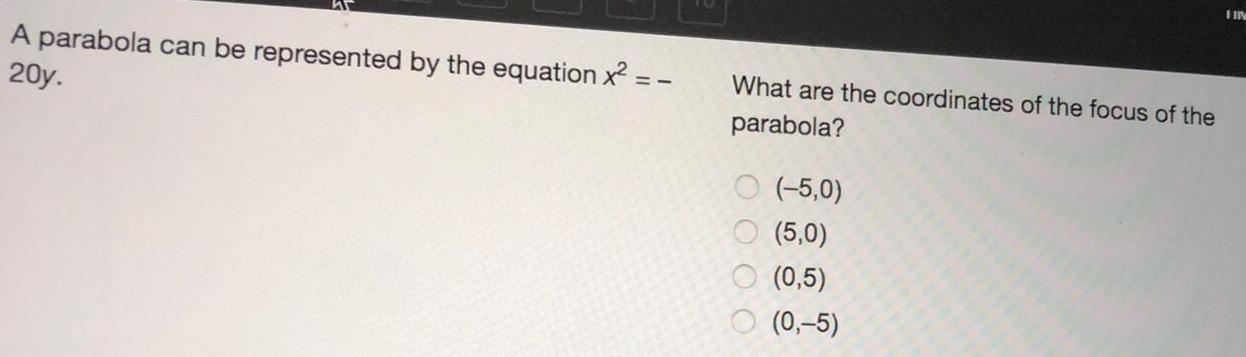Question:

# A parabola can be represented by the equation x² = - 20y.A parabola can be represented by the equation x² = - 20y. What are the coordinates of the focus of the parabola? (-5,0) (5,0) (0,5) (0,-5)